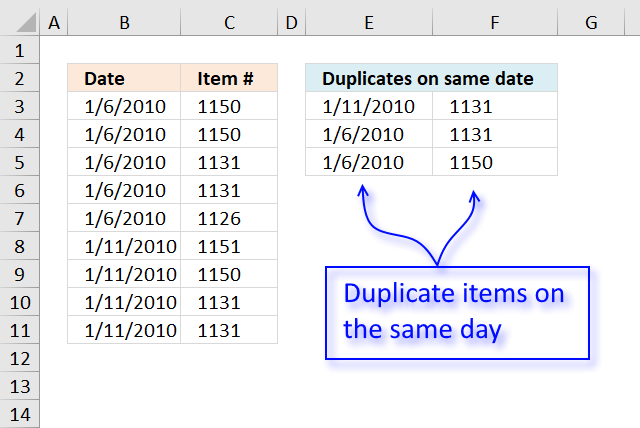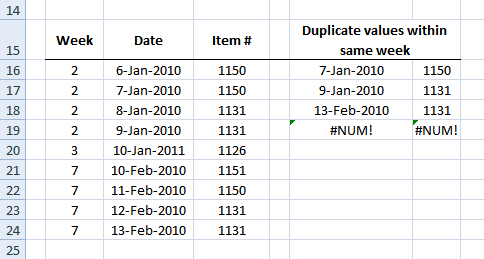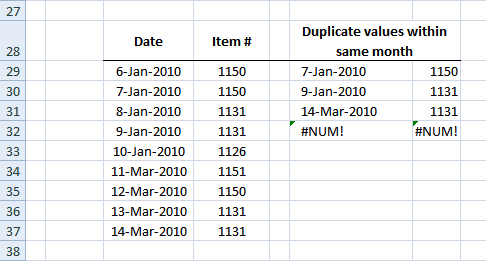Author: Oscar Cronquist Article last updated on December 20, 2018The image above demonstrates a formula in cell E3 that extracts duplicate items if they are on the same date.

Formula in E3:

=LOOKUP(2, 1/((COUNTIFS(\$E\$2:\$E2, \$B\$3:\$B\$11, \$F\$2:\$F2, \$C\$3:\$C\$11)=0)*(COUNTIFS(\$B\$3:\$B\$11, \$B\$3:\$B\$11, \$C\$3:\$C\$11, \$C\$3:\$C\$11)>1)), B\$3:B\$11)

Copy cell and paste it to cell range E3:F5.

### Explaining formula in cell E3

#### Step 1 - Keep track of previous values

The COUNTIFS function calculates the number of cells across multiple ranges that equals all given conditions. If a date and corresponding item already has been displayed the function returns 1. The cell references grow when the cell is copied to cells below, the formula keeps track of previously shown values.

COUNTIFS(\$E\$2:\$E2, \$B\$3:\$B\$11, \$F\$2:\$F2, \$C\$3:\$C\$11)=0

becomes

COUNTIFS("Duplicates on same date",{40184; 40184; 40184; 40184; 40184; 40189; 40189; 40189; 40189},0,{1150; 1150; 1131; 1131; 1126; 1151; 1150; 1131; 1131})=0

becomes

{0;0;0;0;0;0;0;0;0}=0

and returns

{TRUE; TRUE; TRUE; TRUE; TRUE; TRUE; TRUE; TRUE; TRUE}

#### Step 2 - Find duplicates

COUNTIFS(\$B\$3:\$B\$11, \$B\$3:\$B\$11, \$C\$3:\$C\$11, \$C\$3:\$C\$11)>1

becomes

COUNTIFS({40184; 40184; 40184; 40184; 40184; 40189; 40189; 40189; 40189},{40184; 40184; 40184; 40184; 40184; 40189; 40189; 40189; 40189},{1150; 1150; 1131; 1131; 1126; 1151; 1150; 1131; 1131},{1150; 1150; 1131; 1131; 1126; 1151; 1150; 1131; 1131})>1

becomes

{2;2;2;2;1;1;1;2;2}>1

and returns

{TRUE; TRUE; TRUE; TRUE; FALSE; FALSE; FALSE; TRUE; TRUE}

#### Step 3 - Multiply arrays

We use AND logic because both conditions must be met.

(COUNTIFS(\$E\$2:\$E2, \$B\$3:\$B\$11, \$F\$2:\$F2, \$C\$3:\$C\$11)=0)*(COUNTIFS(\$B\$3:\$B\$11, \$B\$3:\$B\$11, \$C\$3:\$C\$11, \$C\$3:\$C\$11)>1)

becomes

{TRUE; TRUE; TRUE; TRUE; TRUE; TRUE; TRUE; TRUE; TRUE}*{TRUE; TRUE; TRUE; TRUE; FALSE; FALSE; FALSE; TRUE; TRUE}

and returns

{1;1;1;1;0;0;0;1;1}

#### Step 4 - Divide 1 with array

The LOOKUP function ignores error values and this is what we are going to use. In order to get an error if a value in the array is FALSE or 0 (zero) we divide 1 with 0 and excel returns !DIV/0 error.

1/((COUNTIFS(\$E\$2:\$E2, \$B\$3:\$B\$11, \$F\$2:\$F2, \$C\$3:\$C\$11)=0)*(COUNTIFS(\$B\$3:\$B\$11, \$B\$3:\$B\$11, \$C\$3:\$C\$11, \$C\$3:\$C\$11)>1))

becomes

1/{1;1;1;1;0;0;0;1;1}

and returns

{1;1;1;1;#DIV/0!;#DIV/0!;#DIV/0!;1;1}

#### Step 5 - Get value

The LOOKUP function returns a value on the corresponding row if it is not an error value, simplified.

LOOKUP(2, 1/((COUNTIFS(\$E\$2:\$E2, \$B\$3:\$B\$11, \$F\$2:\$F2, \$C\$3:\$C\$11)=0)*(COUNTIFS(\$B\$3:\$B\$11, \$B\$3:\$B\$11, \$C\$3:\$C\$11, \$C\$3:\$C\$11)>1)), B\$3:B\$11)

becomes

LOOKUP(2, {1;1;1;1;#DIV/0!;#DIV/0!;#DIV/0!;1;1}, B\$3:B\$11)

becomes

LOOKUP(2, {1;1;1;1;#DIV/0!;#DIV/0!;#DIV/0!;1;1}, {40184; 40184; 40184; 40184; 40184; 40189; 40189; 40189; 40189})

and returns 40189 (1/11/2010) in cell E3.

### Get Excel *.xlsx file

Filter-duplicates-within-same-date-week-month-year.xlsx

### Filter duplicates within same weekFormula in B16:

=WEEKNUM(C16) + ENTER

Array formula in F16:

=INDEX(\$C\$16:\$C\$24, SMALL(IF(MATCH(YEAR(\$C\$16:\$C\$24)&"-"&\$B\$16:\$B\$24&"-"&\$D\$16:\$D\$24, YEAR(\$C\$16:\$C\$24)&"-"&\$B\$16:\$B\$24&"-"&\$D\$16:\$D\$24, 0)<>ROW(\$C\$16:\$C\$24)-MIN(ROW(\$C\$16:\$C\$24))+1, ROW(\$C\$16:\$C\$24)-MIN(ROW(\$C\$16:\$C\$24))+1, ""), ROW(A1))) + CTRL + SHIFT + ENTER.

Copy cell and paste it down as far as needed.

Array formula in G16:

=INDEX(\$D\$16:\$D\$24, SMALL(IF(MATCH(YEAR(\$C\$16:\$C\$24)&"-"&\$B\$16:\$B\$24&"-"&\$D\$16:\$D\$24, YEAR(\$C\$16:\$C\$24)&"-"&\$B\$16:\$B\$24&"-"&\$D\$16:\$D\$24, 0)<>ROW(\$C\$16:\$C\$24)-MIN(ROW(\$C\$16:\$C\$24))+1, ROW(\$C\$16:\$C\$24)-MIN(ROW(\$C\$16:\$C\$24))+1, ""), ROW(A1))) + CTRL + SHIFT + ENTER.

Copy cell and paste it down as far as needed.

### Filter duplicates within same monthArray formula in F29:

=INDEX(\$C\$29:\$C\$37, SMALL(IF(MATCH(YEAR(\$C\$29:\$C\$37)&"-"&MONTH(\$C\$29:\$C\$37)&"-"&\$D\$29:\$D\$37, YEAR(\$C\$29:\$C\$37)&"-"&MONTH(\$C\$29:\$C\$37)&"-"&\$D\$29:\$D\$37, 0)<>ROW(\$C\$29:\$C\$37)-MIN(ROW(\$C\$29:\$C\$37))+1, ROW(\$C\$29:\$C\$37)-MIN(ROW(\$C\$29:\$C\$37))+1, ""), ROW(A1))) + CTRL + SHIFT + ENTER.

Copy cell and paste it down as far as needed.

Array formula in G29:

=INDEX(\$D\$29:\$D\$37, SMALL(IF(MATCH(YEAR(\$C\$29:\$C\$37)&"-"&MONTH(\$C\$29:\$C\$37)&"-"&\$D\$29:\$D\$37, YEAR(\$C\$29:\$C\$37)&"-"&MONTH(\$C\$29:\$C\$37)&"-"&\$D\$29:\$D\$37, 0)<>ROW(\$C\$29:\$C\$37)-MIN(ROW(\$C\$29:\$C\$37))+1, ROW(\$C\$29:\$C\$37)-MIN(ROW(\$C\$29:\$C\$37))+1, ""), ROW(A1))) + CTRL + SHIFT + ENTER.

Copy cell and paste it down as far as needed.

Filter-duplicates-within-same-date-week-month-year.xls
(Excel 97-2003 Workbook *.xls)

IF(logical_test;[value_if:true];[value_if_false])
Checks whether a condition is met, and returns one value if TRUE, and another value if FALSE

SMALL(array,k) returns the k-th smallest row number in this data set.

INDEX(array,row_num,[column_num])
Returns a value or reference of the cell at the intersection of a particular row and column, in a given range

MIN(number1,[number2])
Returns the smallest number in a set of values. Ignores logical values and text

ROW(reference) returns the rownumber of a reference

MATCH(lookup_value;lookup_array; [match_type])
Returns the relative position of an item in an array that matches a specified value

YEAR(serial_number) returns the year of a date, an integer of the range 1900-9999

MONTH(serial_number) returns the month, a number from 1 (January) to 12 (December)

WEEKNUM(serial_number, return_type)
Returns the week number in the year